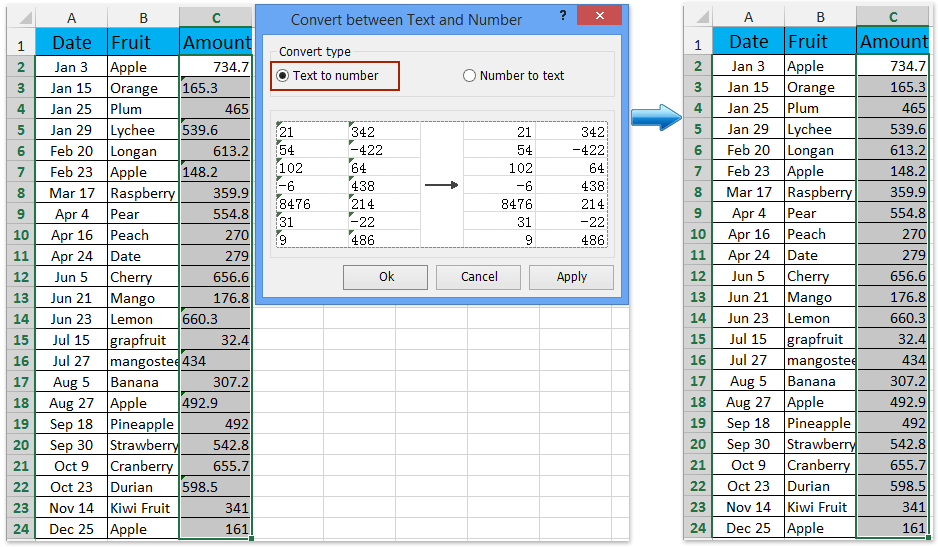## How to sumif with numbers stored as text in Excel?

Generally, we apply the SUMIF function to calculate the total of numbers based on criteria in Excel. But, sometimes the SUMIF function returns 0 because the numbers in the criterion column or evaluated column are stored as text. How to get the correct sumif calculation? Try below methods:

Sumif with numbers in the criterion column stored as text

Sumif with numbers in the evaluated column stored as text

### Easily to convert all numbers stored as text to real numbers or vice versa in Excel

####Sumif with numbers in the criterion column stored as textFor example you have a table as below screenshot, and numbers in the ID column are numbers stored as text. Now you need to sumif IDs are greater than 35000. The normal formula =SUMIF(B2:B24,">35000",C2:C24) will return 0. So how to get correct calculation? Try below method:

Select a blank cell you will place the calculation result, type the formula =SUMPRODUCT(0+(B2:B24>"35000"),C2:C24) into it, and press the Ctrl + Shift + Enter keys at the same time. See screenshot: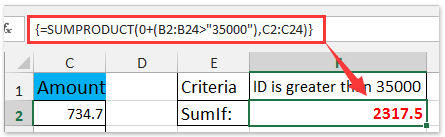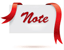Formula is too complicated to remember? Save the formula as an Auto Text entry for reusing with only one click in future! Read more…     Free trial

Note: In above formula, B2:B24 is the criteria column you will sumif based on, 35000 is the specified criteria, and C2:C24 is the evaluated column you will sumif.

####Sumif with numbers in the evaluated column stored as text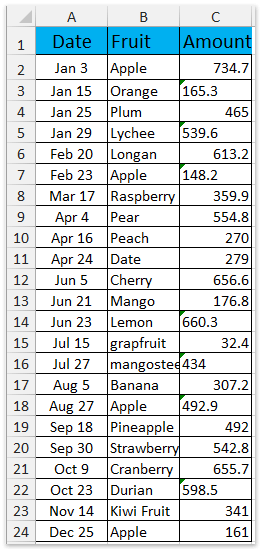Sometimes, the SUMIF function also returns 0 because all or some of numbers in the evaluated column are stored as text. See below screenshot. In this condition, you can get the correct sumif calculation following below method.

Select a blank cell you will place the calculation result in, type the formula =SUMPRODUCT((B2:B24="Apple")*(C2:C24)) into it, and press the Enter key.
And then it returns the correct summing result in selected cell. See screenshot: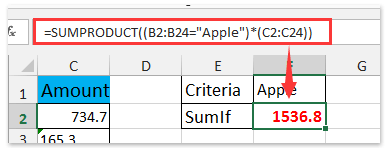Formula is too complicated to remember? Save the formula as an Auto Text entry for reusing with only one click in future! Read more…     Free trial

Notes:

(1) In above formula, B2:B24 is the criteria column you will sumif based on, Apple is the specified criteria, and C2:C24 is the evaluated column you will sumif.

(2) We recommend you apply Kutools for Excel’s Convert between Text and Number utility to quickly convert all numbers stored as text to numbers, and then apply the SUMIF function =SUMIF(B2:B24,"Apple",C2:C24) to get the correct summing result. See screenshot: